# College Algebra Tutorial 15

College Algebra
Tutorial 15: Equations with Rational Expressions

WTAMU > Virtual Math Lab > College Algebra > Tutorial 15: Equations with Rational ExpressionsAnswer/Discussion to 1a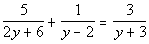Step 1: Simplify by removing the fractions.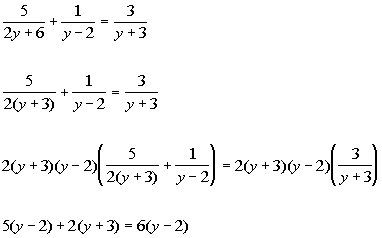*Factor 1st den.

*Mult. both sides by LCD of 2(y + 3)(y - 2)

Step 2: Solve the remaining equation.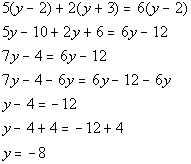*Remove ( ) by using dist. prop.

*Get all y terms on one side

*Inverse of sub. 4 is add. 4

Step 3: Check for extraneous solutions

Note that -8 does not cause any denominators to be zero.  So it is not an extraneous solution.

-8 is the solution to our equation.Answer/Discussion to 1b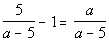Step 1: Simplify by removing the fractions.Step 2: Solve the remaining equation.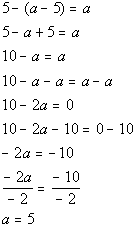*Remove ( ) by using dist. prop.

*Get all a terms on one side

*Inverse of add. 10 is sub. 10

*Inverse of mult. by -2 is div. by -2

Step 3: Check for extraneous solutions

Note that 5 does cause two of the denominators to be zero.

So 5 is an extraneous solution.  That means there is no solution.